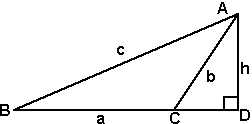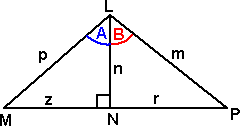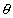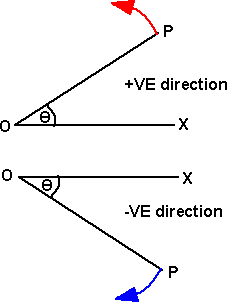# Maths - Trigonometry

## Applications

These functions are very useful in geometry of course. However they are widely used in mathematics, physics and engineering.

## Right Angle Triangle

The sine, cosine and tangent functions represent how the ratio of the sides of a right angled triangle varies with the angle. These can be defined by a right angled triangle in two dimensions as follows:

 Let: a = the length of the edge opposite the right angle b = the length of the edge opposite the angle c = the length of the edge adjacent to the angleThe ratio of these lengths depends on the angle θ and we can define the following functions for each of the ratios:

 sine function sin(θ) = b / a cosine function cos(θ) = c / a tangent function tan(θ) = b / c cosecant cosec(θ) = a / b secant sec(θ) = a / c cotangent cot(θ) = c / b

these functions are related to each other as follows:

tan(θ) = sin(θ) / cos(θ)

cosec(θ) = 1 / sin(θ)

sec(θ) = 1 / cos(θ)

cot(θ) = 1 / tan(θ)

## Terminology and Notation

### The angle

 The angle being considered is usually denoted by the greek letter theta: θ. We can also denote the angle by three points which define that angle, for example in the above diagram: θ = ABC Where the middle point is where the angle is measured between the other two points.### The sides

The sides can be denoted in different ways:

• we often use the names: hypotenuse, opposite and adjacent.
• we could use the letters a,b and c
• we could use the upper case letters for the two points the line connects: AB, BC and AC.
 hypotenuse - the side opposite the right angle a BC opposite - the side opposite the angle being considered b AC adjacent - the side adjacent to the angle being considered c AB

### The Vertices

The points of the triangle, the vertices, are here denoted by upper case letters A,B and C

### The Trig Functions

The main trigometric functions are: sine, cosine and tangent. These are often abbreviated to sin, cos and tan.

There are three others cosecant, secant and cotangent . They not used so often because they are the reciprocal of sine, cosine and tangent. They are abbreviated to cosec, sec and cot.

## Trig Identities

The values of sin, cos and tan are related to each other, for instance,

• tan A = sin A / cos A
• cos2A + sin2A = 1

These 'identities' can be proved by using the sides of a right angled triangle as follows:

tan A = b / c = (b / a) / (c / a) = sin A/cos A

From Pythagoras we know:

b2 + c2 = a2

dividing both sides by a2 gives:

b2 / a2 + c2 / a2 = 1

cos2A + sin2A = 1

## Complimentary and Supplementary Angles

Two angles whose sum is 90° are said to be complimentary, each is said to be the compliment of the other. Where the sum is 180° the angles are said to be supplementary.sin(θ - 90°) = BA / BC = cos(θ) cos(θ - 90°) = AC / BC = sin(θ) tan(θ - 90°) = AC / AB = cot(θ)

## Non-Right Angled Triangles - The Sine Rule

We started with right angled triangles but we can also use trig functions for other triangles:

### Acute angled triangle

 Drop a perpendicular from A to BC and let AD = h, then, sin B = h / c h = c sin B similarly, sin C = h / b h = b sin Ccombining these gives:

c sin B = b sin C

so,

b / sin B = c / sin C

similarly if we put a perpendicular from B onto AC we can show that:

a / sin A = c / sin C

So, combining these, we get:

a / sin A = b / sin B = c / sin C

where:

• sin A = the sine of the angle at corner A
• sin B = the sine of the angle at corner B
• sin C = the sine of the angle at corner C
• a = length of side a
• b = length of side b
• c = length of side c

### Obtuse angled triangle

 This time we need to extend the line from B to C onto D so that it can meet a perpendicular from A. sin B = h / c h = c sin B similarly, sin ACD = h / b h = b sin ACDcombining these gives:

c sin B = b sin ACD

but sin ACD = sin(180° - ACD) = sin c

so,

b / sin B = c / sin C

similarly if we put a perpendicular from B onto AC we can show that:

a / sin A = c / sin C

So, combining these, we get:

a / sin A = b / sin B = c / sin C

where:

• sin A = the sine of the angle at corner A
• sin B = the sine of the angle at corner B
• sin C = the sine of the angle at corner C
• a = length of side a
• b = length of side b
• c = length of side c

#### Example 1

solve triangle ABC if:

• A = 70°
• C = 58°16'
• b = 6m

Angles of triangle add up to 180° so,

B = 180° - (70° + 58°16')

B = 51°74'

since: a / sin A = b / sin B then,

a = b * sin A/ sin B

a = 6m * sin 70°/ sin 51°74'

a = 6.63m

#### Example 2

solve triangle ABC if:

• c = 85.3
• b = 70.25
• B = 40°

b / sin B = c / sin C

sin C = sin B * c / b

C = 51°44'

however this is an ambiguous case since there is also a solution at sin(180°-51°19')

## Non-Right Angled Triangles - The Cosine Rule

 We can use this to solve for triangles where the sine rule is not applicable (when we know 2 sides and the included angle). We create a perpendicular to the side b, mark the point this joins as D.We can apply Pythagoras to the two inner triangles:

h2 = c2 - n2

a2 = (b-n)2 - h2

expanding the second one, and substitute the first for h, gives:

a2 = b2 - 2bn + n2+ c2 - n2

a2 = b2 - 2bn + c2 but n = c cos A which gives,

a2 = b2 + c2 - 2*b*c*cos A

we can do similar constructions based on the other sides:

b2 = c 2 + a 2 - 2*c*a*cos B

c2 = a 2 + b 2 - 2*a*b*cos C

rearranging these equations gives:

cos A = (b2 + c2 - a2)/2*b*c

cos B = (c2 + a 2 - b 2)/2*c*a

cos C = (a2 + b 2 - c 2)/2*a*b

## Area of triangles

The area of a triangle is: 1/2 base * perpendicular height

 therefore: area = 1/2 a * h but h = c*sin B therefore, area = 1/2 a * c*sin Bsimilarly we can do the same construction for the other sides:

area = 1/2 a * b*sin C

area = 1/2 b * c*sin A

This is half the product of two sides and the sin of the included angle.

We want to express sin(A+B) in terms of trig ratios

#### sin(A+B)

 From the diagram we can see that the area of the whole is equal to the sum of the areas of the inner triangles: area LMP = area LMN + area LNP 0.5 p m sin(A+B) = 0.5 n p sin A + 0.5 m n sin Bwhich gives:

sin(A+B) = (n/m) sin A + (n/p) sin B

but n/m = cos B and n/p = cos A

substituting these gives:

sin(A+B) = sin A cos B + cos A sin B

#### cos(A+B)

apply cos rule:

cos(A+B) = (p2 + m2 - (q+r)2)/2*m*p

cos(A+B) = (p2 + m2 - q2- 2*q*r- r2)/2*m*p

cos(A+B) = ((p2 - q2)+(m2 - r2)- 2*q*r) / 2*m*p

cos(A+B) = (n2+n2- 2*q*r) / 2*m*p

cos(A+B) = (n2- q*r) / m*p

cos(A+B) = n2/m*p - q*r/m*p

cos(A+B) = n/p * n/m - q/p * r/m

cos(A+B) = cos A cos B - sin A sin B

#### tan(A+B)

tan(A+B) = sin(A+B)/cos(A+B)

tan(A+B) = (sin A cos B + cos A sin B)/(cos A cos B - sin A sin B)

divide top & bottom by cos A cos B

tan(A+B) = ((sin A cos B/cos A cos B) + (cos A sin B/cos A cos B))/((cos A cos B/cos A cos B) - (sin A sin B/cos A cos B))

tan(A+B) = ((sin A /cos A) + (sin B/cos B))/(1 - (sin A sin B/cos A cos B))

tan(A+B) = (tan A + tan B)/(1 - tan A tan B)

### Double Angle Formulae

from addition formulae if A=B

• sin(2A) = 2 sin A cos A
• cos(2A) = cos2A - sin2A = 1 - 2*sin2A
• tan(2A) = 2 tan A/(1 - tan2A)

The second form of the cos(2A) formula can be proved as follows:

from the addition formulae if A=B we get:

cos(2A) = cos2A - sin2A

but we can combine this with the identity:

cos2A + sin2A = 1

substituting

cos(2A) = (1 - sin2A) - sin2A

cos(2A) = 1 - 2*sin2A

I have put further information about double angle and half angle formulae on the page here.

### Subtraction formulae

from addition formulae if A=B

• sin(A-B) = sin A cos B - cos A sin B
• cos(A-B) = cos A cos B + sin A sin B
• tan(A-B) = (tan A - tan B)/(1 + tan A tan B)

## Angles of Any Magnitude

So far we have defined the trig functions using right angled triangles and therefore used angles between 0 and 90 degrees however we can extend the concept of sine, cosine, etc. and use angles beyond that range. If we plot the angle () across (left to right) and the ratio of sides up (bottom to top) then we get a wave-like function like this which repeats continuously in both directions:### Angle Sign

Ifis formed by OP rotating about O and rotation is anticlockwiseis said to be positive. If clockwiseis said to be negative.### Results

Because the trig functions repeat like this, we can derive the values outside 0°-90° from the values inside the 0°-90° range.

• sin(180° - θ) = sin(θ)
• sin(180° + θ) = -sin(θ)
• cos(90° + θ) = -sin(θ)
• cos(90° - θ) = sin(θ)
• tan(270° + θ) = -cotan(θ)
• tan(270° - θ) = cotan(θ)
• sec(180° + θ) = -sec(θ)
• cosec(180° - θ) = cosec(θ)
• cot(360° + θ) = cot(θ)
• sin(360° - θ) = -sin(θ)
• cos(270° + θ) = sin(θ)
• cos(270° - θ) =In the first quadrant, angles between 0° and 90°, both the x axis and the y axis are positive, therefore the ratios are positive, and all the trig functions are positive.

In the second quadrant, angles between 90 ° and 180°, the x axis is negative and the y axis is positive, therefore:

 sine function sin(θ) = b(+ve) / a(+ve) = (+ve) cosine function cos(θ) = c(-ve) / a(+ve) = (-ve) tangent function tan(θ) = b(+ve) / c(-ve) = (-ve) cosecant cosec(θ) = a(+ve) / b(+ve) = (+ve) secant sec(θ) = a(+ve) / c(-ve) = (-ve) cotangent cot(θ) = c(-ve) / b(+ve) = (-ve)

In the first quadrant, angles between 180° and 270°, both the x axis and the y axis are negative, therefore:

 sine function sin(θ) = b(-ve) / a(+ve) = (-ve) cosine function cos(θ) = c(-ve) / a(+ve) = (-ve) tangent function tan(θ) = b(-ve) / c(-ve) = (+ve) cosecant cosec(θ) = a(+ve) / b(-ve) = (-ve) secant sec(θ) = a(+ve) / c(-ve) = (-ve) cotangent cot(θ) = c(-ve) / b(-ve) = (+ve)

 metadata block see also: Correspondence about this page Book Shop - Further reading. Where I can, I have put links to Amazon for books that are relevant to the subject, click on the appropriate country flag to get more details of the book or to buy it from them.Mathematics for 3D game Programming - Includes introduction to Vectors, Matrices, Transforms and Trigonometry. (But no euler angles or quaternions). Also includes ray tracing and some linear & rotational physics also collision detection (but not collision response). Other Math Books